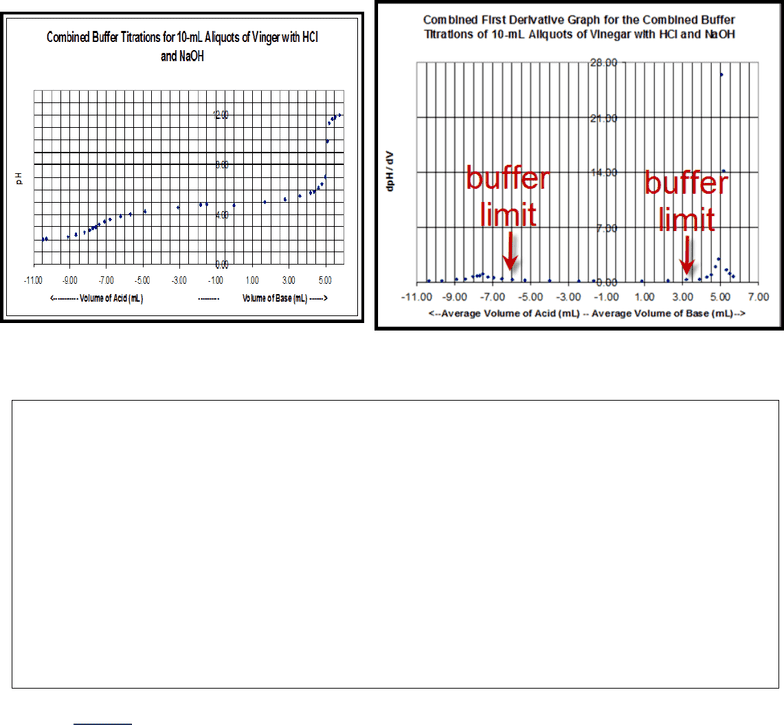Textbook Notes (290,000)
US (110,000)
UCLA (2,000)
CHEM (200)
Chapter 5

# CHEM 14BL Chapter Notes - Chapter 5: Acid Dissociation Constant, Buffer Solution, Conjugate Acid

Department
Chemistry and Biochemistry
Course Code
CHEM 14BL
Professor
Henary Maher
Chapter
5

This preview shows half of the first page. to view the full 3 pages of the document.Buffer Post Lab
Combined Buffer Titration Curve (and its First Derivative)
Example: Using the literature pKa value for acetic acid (i.e., 4.74) and the pH of the solution at
that point of the titration, calculate the ratio of [OAc-]/[HOAc] at the buffer limit for HCl and
NaOH (using the 10-mL aliquot graph shown above). You may assume that the Henderson-
Hasselbach equation can be used in this region.
*In the combined buffer titration graph (and its first derivative) the volumes to the left of the origin correspond to
volume of acid added; volumes to the right of the origin correspond to base added. Be careful to not get confused
the negative signs DO NOT suggest negative amounts of volume of acid.
How do you determine the buffer limits on the combined buffer first derivative graph (Show on the graph)?
In this graph, determine the buffer limits by looking where there is a first sudden increase in the dpH/dV. You
can look at the graph above as an example.
What happens to the ratio of [A-]/[HA] as:
Acid is added? The ratio becomes smaller; [CH3COOH] > [CH3COO-]
Base is added? The ratio becomes larger; [CH3COO-] > [CH3COOH]
What is the relationship between buffer and buffer capacity?
The more (amount of) buffer you have, the larger the buffer capacity.